## Subfactorial

The number of Permutations ofobjects in which no object appear in its natural place (i.e., so-called Derangements'').(1)

or(2)

where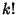is the usual Factorial and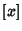is the Nint function. The first few values are,,,,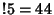,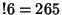,,, ... (Sloane's A000166). For example, the only Derangements ofare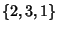and, so. Similarly, the Derangements ofare,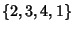,,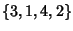,,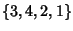,,, and, so.

The subfactorials are also called the Rencontres Numbers and satisfy the Recurrence Relations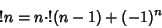(3)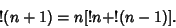(4)

The subfactorial can be considered a special case of a restricted Rooks Problem.

The only number equal to the sum of subfactorials of its digits is(5)

References

Dörrie, H. §6 in 100 Great Problems of Elementary Mathematics: Their History and Solutions. New York: Dover, pp. 19-21, 1965.

Madachy, J. S. Madachy's Mathematical Recreations. New York: Dover, p. 167, 1979.

Sloane, N. J. A. Sequence A000166/M1937 in An On-Line Version of the Encyclopedia of Integer Sequences.'' http://www.research.att.com/~njas/sequences/eisonline.html and extended entry in Sloane, N. J. A. and Plouffe, S. The Encyclopedia of Integer Sequences. San Diego: Academic Press, 1995.

Stanley, R. P. Enumerative Combinatorics, Vol. 1. Cambridge, England: Cambridge University Press, p. 67, 1997.